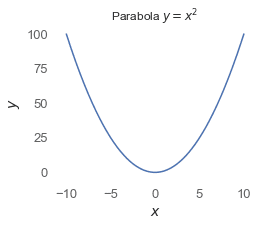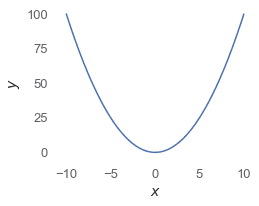search
Search
Unlock 100+ guides
search toc
close
Cancel
Post
account_circle
Profile
exit_to_app
Sign out
search
keyboard_voice
close
Searching Tips
Search for a recipe:
"Creating a table in MySQL"
Search for an API documentation: "@append"
Search for code: "!dataframe"
Apply a tag filter: "#python"
Useful Shortcuts
/ to open search panel
Esc to close search panel
to navigate between search results
d to clear all current filters
Enter to expand content previewDoc SearchCode Search BetaSORRY NOTHING FOUND!
mic
Start speaking...Voice search is only supported in Safari and Chrome.
Shrink
Navigate to
check_circle
Mark as learned
thumb_up
0
thumb_down
0
chat_bubble_outline
0
Comment
auto_stories Bi-column layout
settings

# Drawing a function in Matplotlib

schedule Aug 11, 2023
Last updated
local_offer
PythonMatplotlib
Tags
expand_more

# Drawing a parabola

To draw a parabola (`y=x**2`):

``` import matplotlib.pyplot as pltx = np.linspace(-10, 10, 1000) # Domain from -10 to 10 with 1000 data-pointsplt.title("Parabola \$y=x^2\$")plt.xlabel("\$x\$", fontsize=14)plt.ylabel("\$y\$", fontsize=14)plt.plot(x, x**2) ```

The output is as follows:# Helper function to draw functions

``` def my_func(x): return x**2def plot_function(x_lim, my_func): """ @param x_lim {Array} an array of size two that contains the lower and upper x limits @param my_func {Function} the function that we want to plot """ x = np.linspace(x_lim, x_lim, 1000) plt.xlabel("\$x\$", fontsize = 14) plt.ylabel("\$y\$", fontsize = 14) plt.plot(x, my_func(x)) ```

We can use plot a curve like so:

``` plot_function([-10,10], my_func) ```

The output is as follows:thumb_up
thumb_down
Comment
Citation
Ask a question or leave a feedback...
thumb_up
0
thumb_down
0
chat_bubble_outline
0
settings
Enjoy our search
Hit / to insta-search docs and recipes!